## Central Tendency Worksheet (Mac Version)

Find the mean, median, and mode values for each of the following four sets of data.

To do this work we will employ the same strategy that we have used in earlier examples:
1. Insert the USB drive
2. Create a new folder (directory) to hold our work
3. Copy copy our `model.R` file to the new directory
4. Rename the new file
5. Start RStudio by double clickng on the new file
6. Put the desired commands into the editor pane
7. Save that that work in the file
8. Perform the commands by highlighting the commands that we want to run, then pressing the `Run` icon.
9. Observe the results in the Console pane
10. Finally, close RStudio
11. Safely remove the USB drive

Let us start the process.

Insert the USB drive. This, after a moment or two, opens the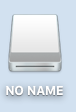icon on the desktop. If we click on that we cause the Finder to open a window such as the one shown in Figure 1.

Figure 1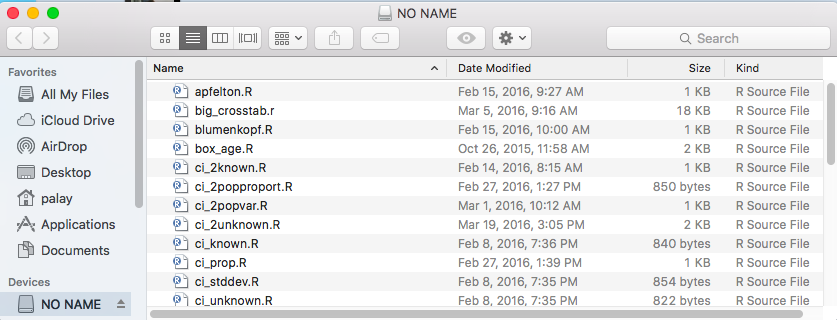Click on the Finder menu option File to open the window shown in Figure 1.5.

Figure 1.5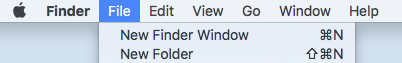Click on the `New Folder` option to get Figure 2.

Figure 2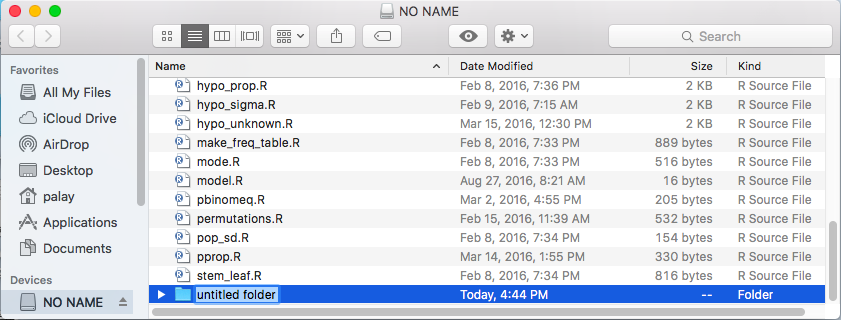Rather than use the default folder name, `Nuntitled folder` of Figure 2, type in a new name. For this project I used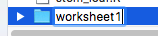.

Once the folder has been created and renamed, we locate the `model.R` file in the list, point to it and right click to open the window shown in Figure 3.

Figure 3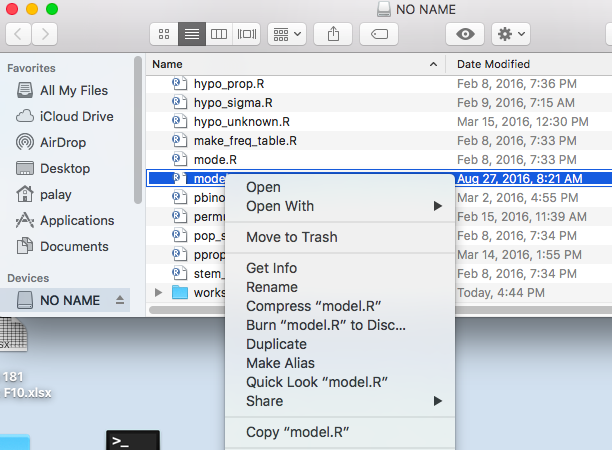In that window we select the `Copy "model.R"` option.

After that we return to find our `worksheet1` directory and we double click on it to open the newly created empty directory shown in Figure 4.

Figure 4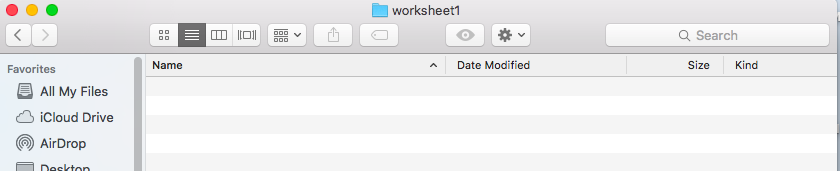Then point to an open area, right click, and you should see the small window in Figure 5.

Figure 5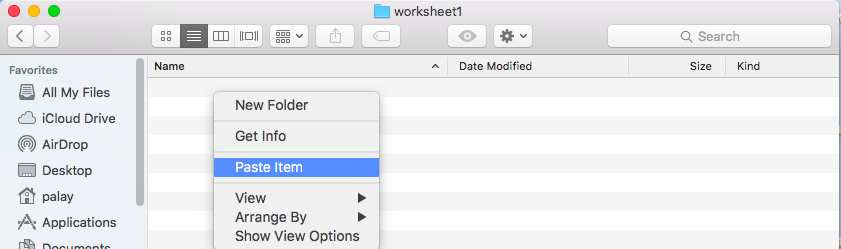There you want to select the `Paste` option to get the copy of the file put into our new directory. We can rename the file (click on it once, wait a second, and click again to highlight the name of the file) as shown in Figure 6.

Figure 6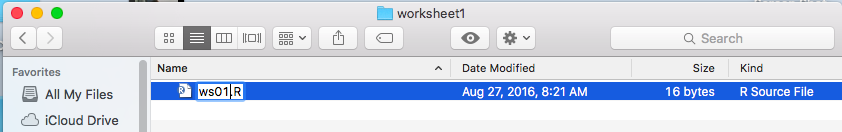We ctype the new name, `ws01.R` as shown in Figure 7.

Figure 7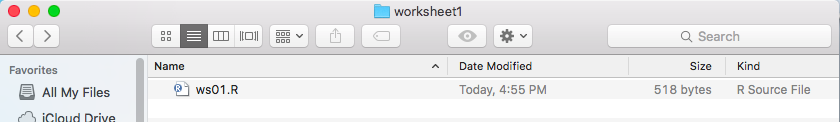Once that is in place we can double click on the new file name to open RStudio, shown in Figure 8.

Figure 8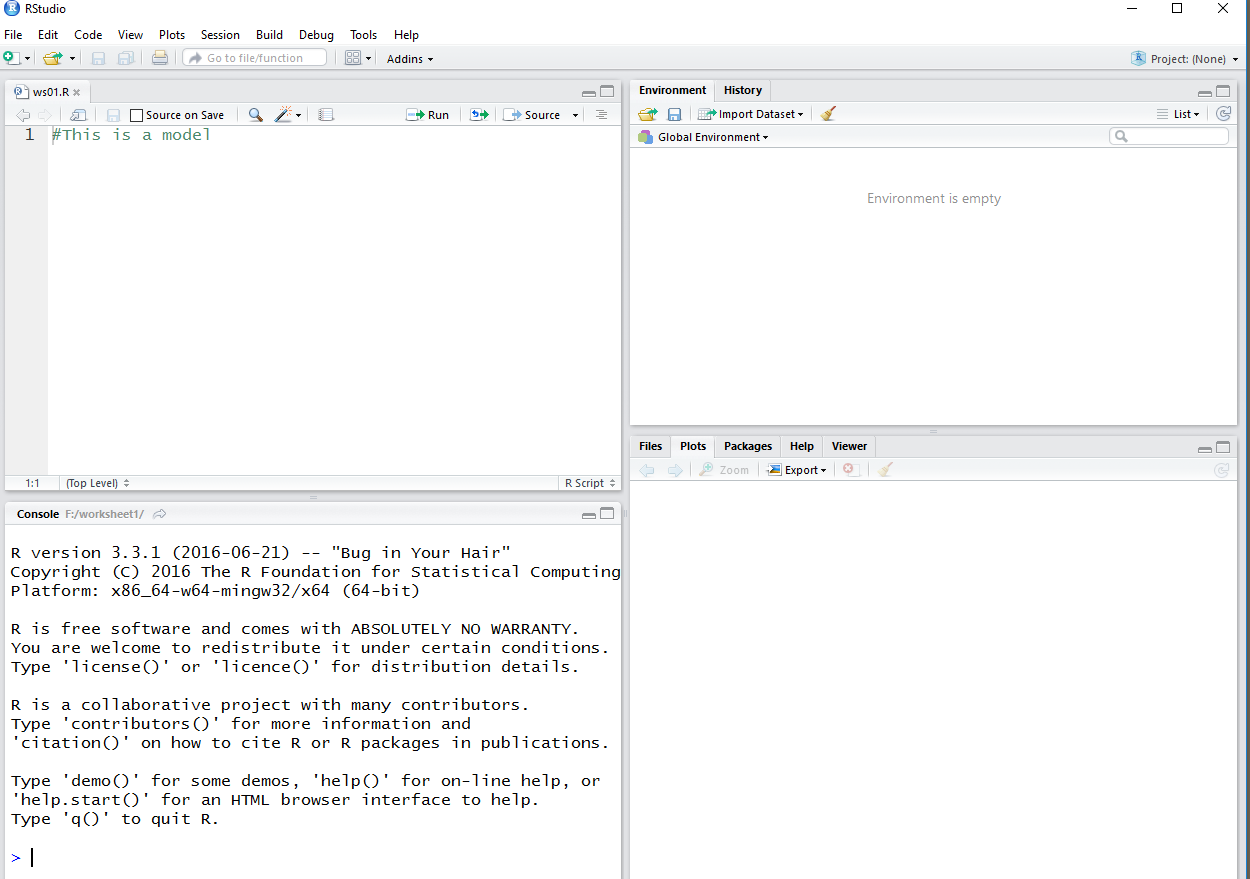A closer examination of the Editor pane is shown in Figure 9. This is just what we expect since we know this to be the contents of the original `modl.R` file.

Figure 9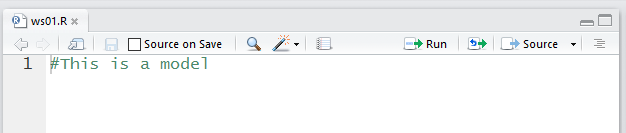However, we want different commands.

The commands that we want are:
```   # commands to do work sheet 1
# first we will load the required functions
source("../gnrnd4.R")
source("../mode.R")
# Then we need to generate each set of data and
# follow that with the commands to find the
# mean, median, and mode of that data.
gnrnd4(key1=45638703,key2=1800462)
L1
mean(L1)
median(L1)
Mode(L1)
gnrnd4(key1=291659302,key2=1400059)
L1
mean(L1)
median(L1)
Mode(L1)
gnrnd4(key1=604729501,key2=1100326)
L1
mean(L1)
median(L1)
Mode(L1)
gnrnd4(key1=520079004,key2=400135)
L1
mean(L1)
median(L1)
Mode(L1)

```
Figure 10 shows the Editor when it had the first 17 of those lines in it.

Figure 10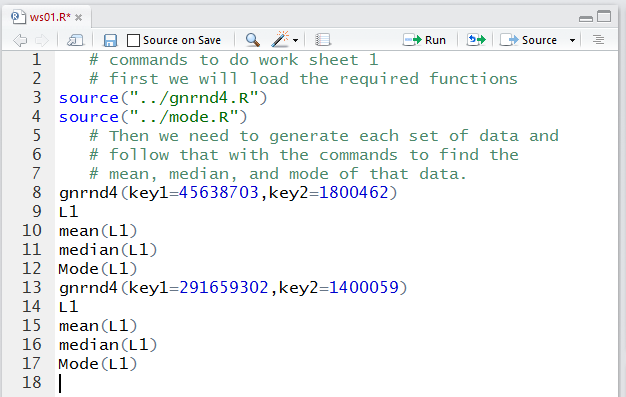Figure 11 shows the Editor pane after all the lines have been entered.

Figure 11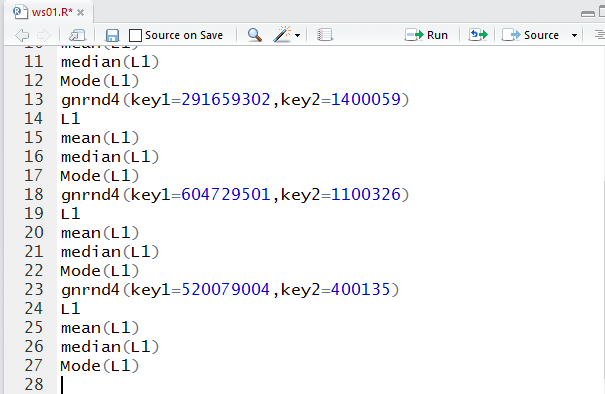We notice that the name of the file, `ws01.R` shown in the file tab is displayed in red. That is an indication that the contents of the file have yet to be saved. We click on the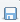icon to save the file. That will change the file name to be black, as we see in Figure 12.

Figure 12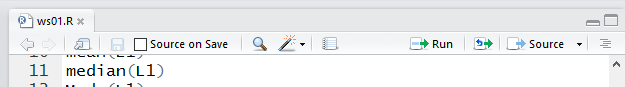If we turn our attention to the lower right pane in the RStudio window, and if we click on the `Files` tab there, we will see that our file is there and that it holds the 518 bytes that we put into it. This is shown in Figure 13. You should notice that a second file also shows up. That is the `.Rhistory` file that R creates. It is a "hidden" file. We will not see it in the Finder view of the folder.

Figure 13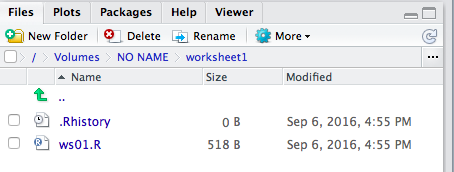Now we are ready to run all of the commands that we prepared. We could highlight and run all of the commands in the Editor pane, but if we did so we would have to scroll through the Console display to see all of the results. Instead, here, we will select just the commands that we want to see at each step of the process.

Figure 14 shows that we can highlight the commands that load our functions (lines 3 and 4) and the commands to generate, view, and find the answers for the data in table 1.

Figure 14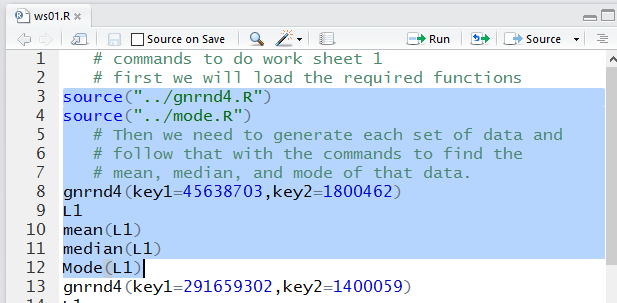Once that is highlighted, we click on the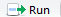icon to have those commands performed. We can see the result in the Console pane, shown in Figure 15.

We should verify that the values shown for L1 in Figure 15 are identical to the values we were given in  1.

Figure 15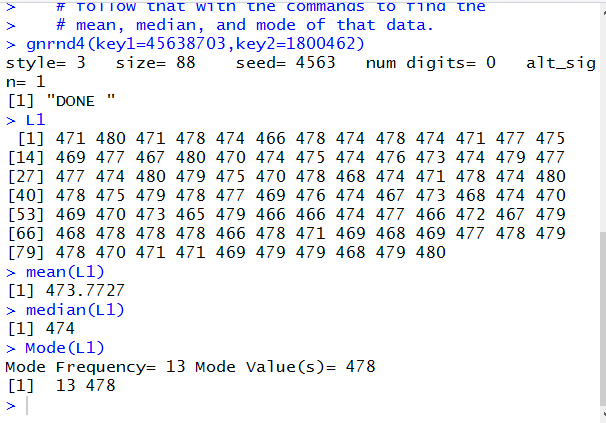Then, reading the rest of Figure 15, w see that the mean is 473.7727, the median is 474, and the mode is 478, a value that appears 13 times in the data.

We should also note in the Environment pane, Figure 16, that we have the variables and the functions that we expect to be defined.

Figure 16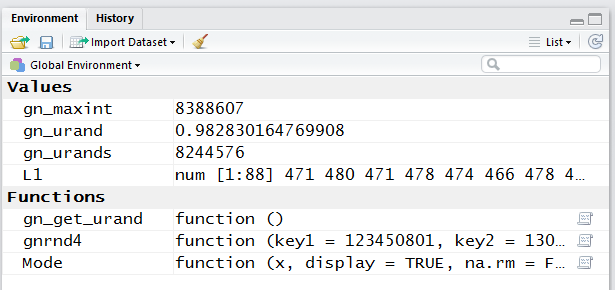Now we are ready to work with the data in Table 2. Therefore, we highlight the appropirate commands in the Editor pane (lines 13 through 17), as shown in Figure 17. Then press theicon.

Figure 17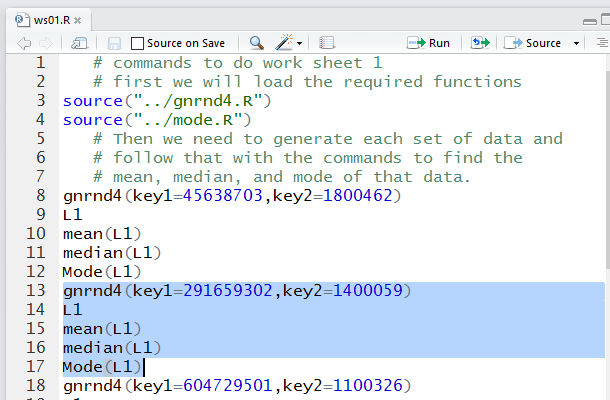Figure 18 shows the results of thos commands. Again we verify that the values displayed are identical to those in Table 2. And we find that the mean is 63.56383, the median is 63, and the mode is 59, a value that appears 16 times in the data.

Figure 18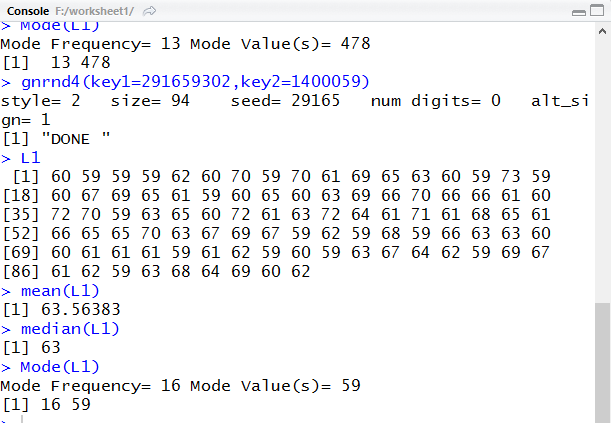Returning to the Editor pane we highlight the next set of commands.

Figure 19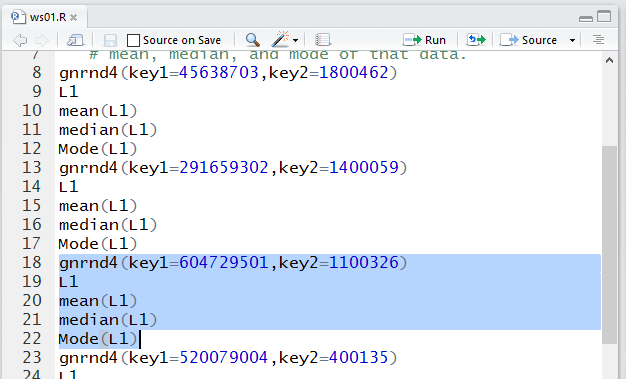Then we run them to get Figure 20.

Figure 20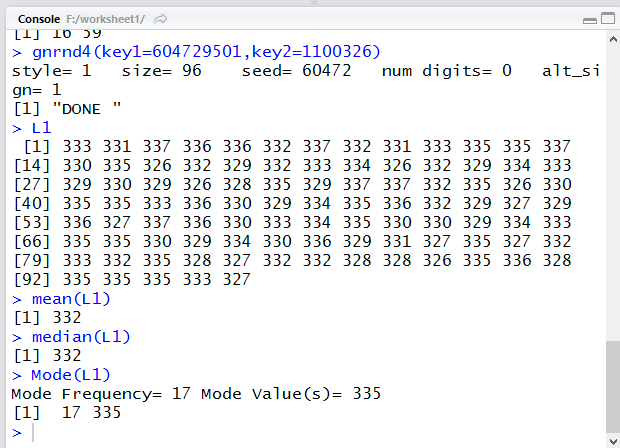Again we verify that the values displayed are identical to those in Table 3. And we find that the mean is 332, the median is 332, and the mode is 335, a value that appears 17 times in the data.

That leaves just the last set of commands to highlight, as shown in Figure 21.

Figure 21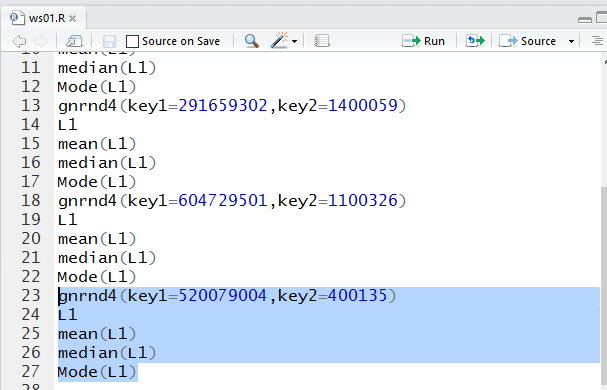Then we run them to get Figure 22.

Figure 22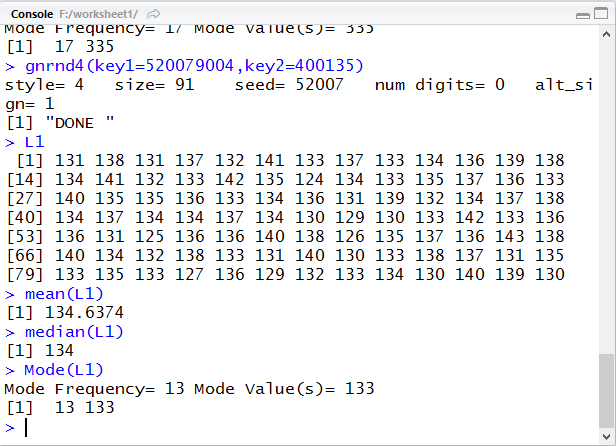Again we verify that the values displayed are identical to those in Table 4. And we find that the mean is 134.6374, the median is 134, and the mode is 133, a value that appears 13 times in the data.

We have found all the values we needed to find. Therefore, we can quit R via the `q()` command shown in Figure 23.

Figure 23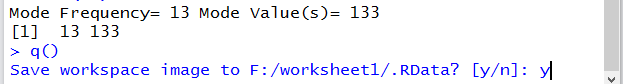Once we have left RStudio, we can see, in Figure 24, the Finder shows that we have just one file in our directory. Again, that is because the Mac hides files with names that start with a period.

Figure 24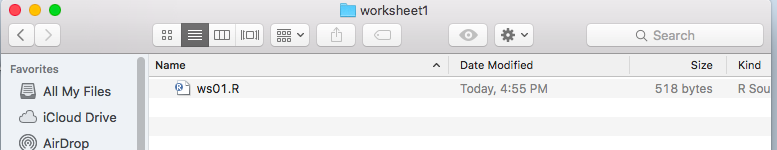If we werre to restart RStudio, by double clcking on the `ws01.R` file in Figure 24, then we woud see, in the `Files` tab, all three files, as is shown in Figure 24.5

Figure 24.5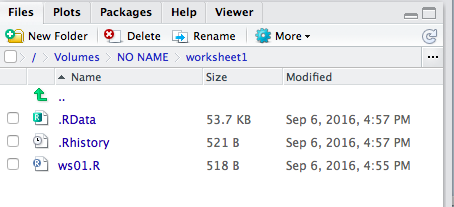All that is left to do is to safely remove the USB drive. We close the Finder window that is looking at our directory. Then, pointing to the drive icon,, we can right click to open the menu shown in Figure 25.

Figure 25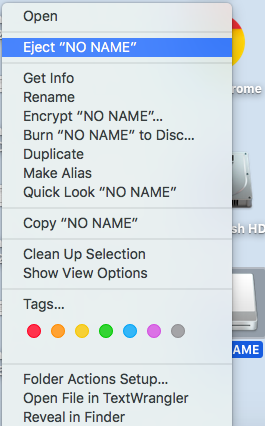There we click on the `Eject "NO NAME"` option. Once that is done, eventually, the USB drive icon on the desktop will disappear. At that point we can safely remove the USB drive.